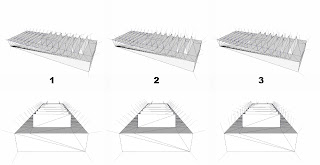## Friday, April 10, 2009

### On the computation of vertex normals

Computing per-vertex normal is usually a rather neglected task. There is a very popular solution that is usually considered reasonable and good for all purposes, until you hit some nasty counter-examples... Short summary of the most common approaches:
1. Compute an area weighted average of the normals of all the faces incident on the vertex. This is the classical approach, very handy, just because if you compute your face normals using a simple cross product between two edges of a triangle, you get for free a normal vector whose length is twice the triangle area. So just summing the un-normalized cross products gives you the right weights. Referred many many times as THE method for computing per vertex normals.
2. Compute an angle weighted average of the normals of all the faces incident on the vertex. Probably first seen on . Mathematically sound, in the sense that it catch the limit behavior of the surface in a local neighborhood of the vertex. Simple, but it requires some trigonometric computations, so it is usually neglected by hard core optimization fans.
3. Use the "Mean Weighted by Sine and Edge Length Reciprocal" proposed by N. Max . One of the many possible variations of smart weighting with the nice property of NOT using trigonometric computations.
Without going into gory details (that you can find in ), you should know that the classical approach can give rise to some VERY counter-intuitive normals. Below a practical example of the difference between the above three algorithms. Note how the direction of the normals on the top of the thin up-wedges is strongly biased by the underlying tessellation. Yes this is a rather badly triangulated nasty example, but this stuff happens.Just for fun (and to overcome a bug in another algorithm) we have added the three explicit methods for computing normals in the latest beta of MeshLab. Personal, un-scientific, subjective feelings:
1. simple but dangerous
2. good
3. almost good

 G. Thurmer, C. A. Wuthrich, "Computing vertex normals from polygonal facets"
Journal of Graphics Tools, 3 1998
 Nelson Max, "Weights for Computing Vertex Normals from Facet Normals", Journal of Graphics Tools, 4(2) (1999)
 S. Jin, R.R. Lewis, D. West, "A comparison of algorithms for vertex normal computations", The Visual Computer, 2005 - Springer